Скачать презентацию Formal Methods for System Integration Michael Fourman Informatics

4b9d56fa6336a6e65d6029e810dbe670.ppt

• Количество слайдов: 24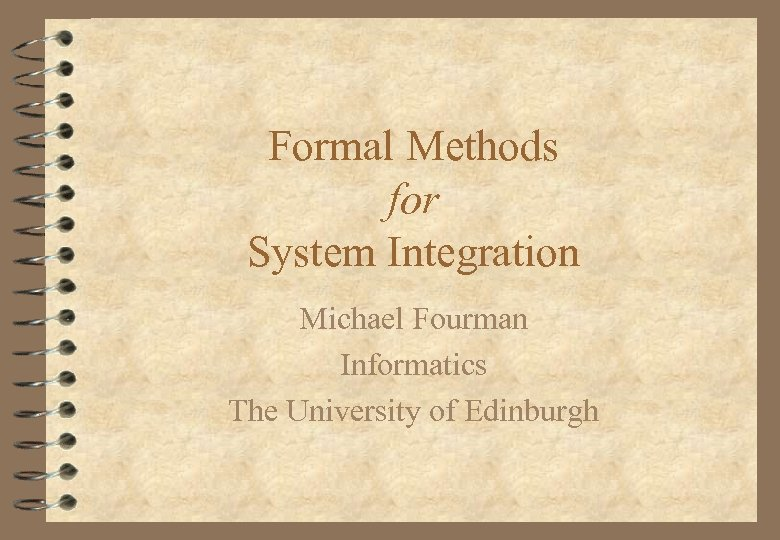Formal Methods for System Integration Michael Fourman Informatics The University of Edinburgh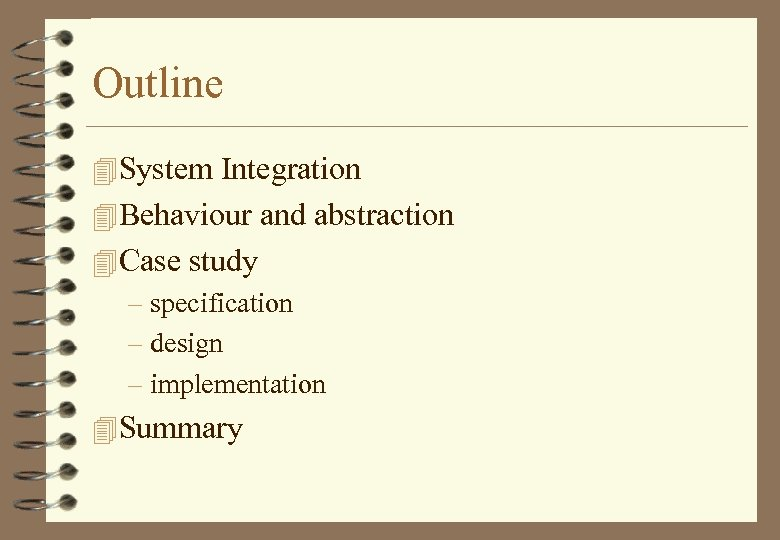Outline 4 System Integration 4 Behaviour and abstraction 4 Case study – specification – design – implementation 4 Summary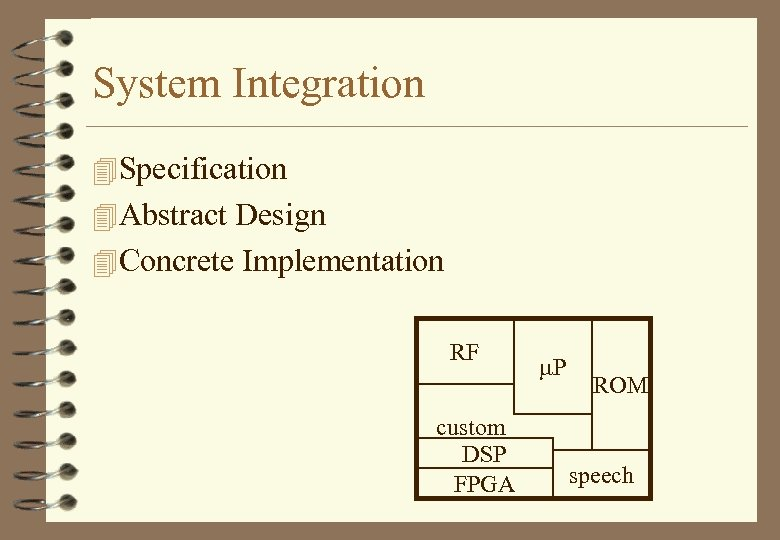System Integration 4 Specification 4 Abstract Design 4 Concrete Implementation RF custom DSP FPGA m. P ROM speech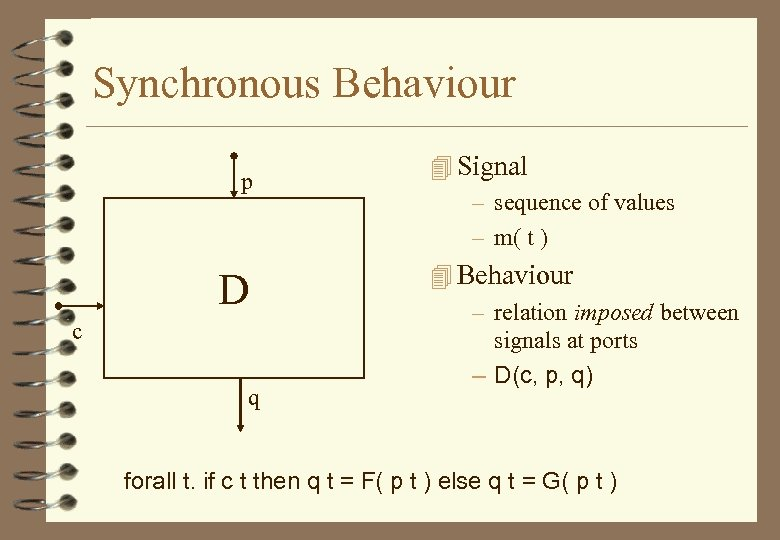Synchronous Behaviour p D c q 4 Signal – sequence of values – m( t ) 4 Behaviour – relation imposed between signals at ports – D(c, p, q) forall t. if c t then q t = F( p t ) else q t = G( p t )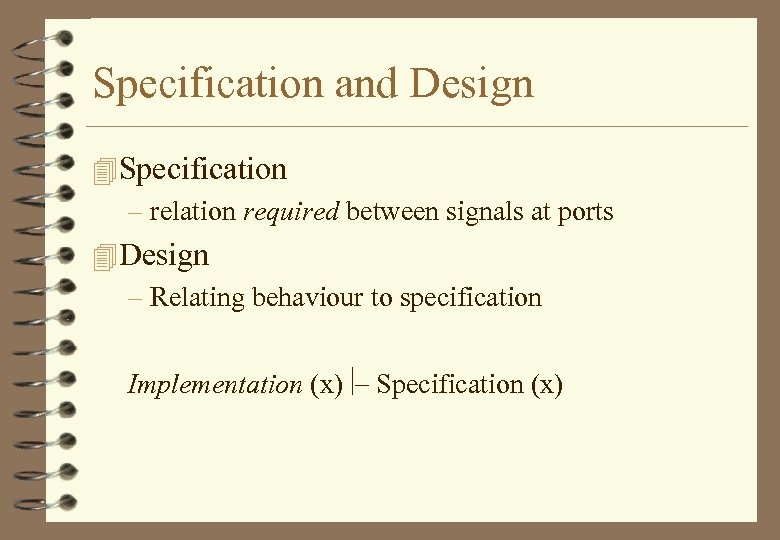Specification and Design 4 Specification – relation required between signals at ports 4 Design – Relating behaviour to specification Implementation (x)ú- Specification (x)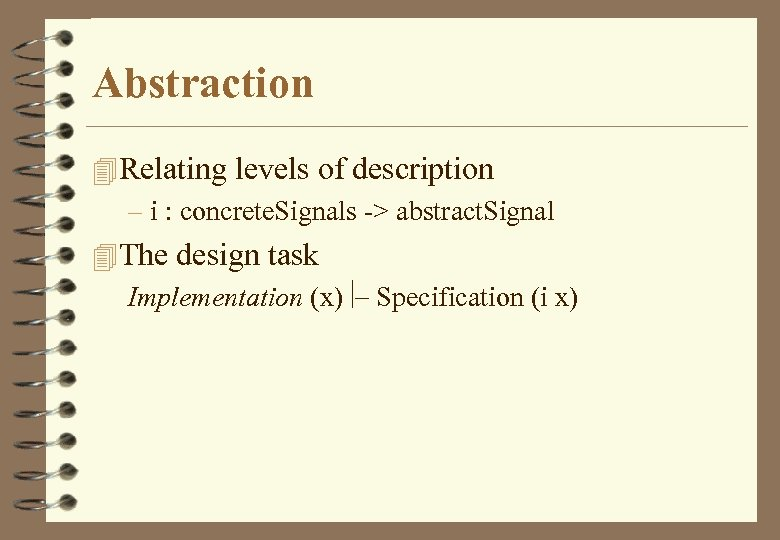Abstraction 4 Relating levels of description – i : concrete. Signals -> abstract. Signal 4 The design task Implementation (x)ú- Specification (i x)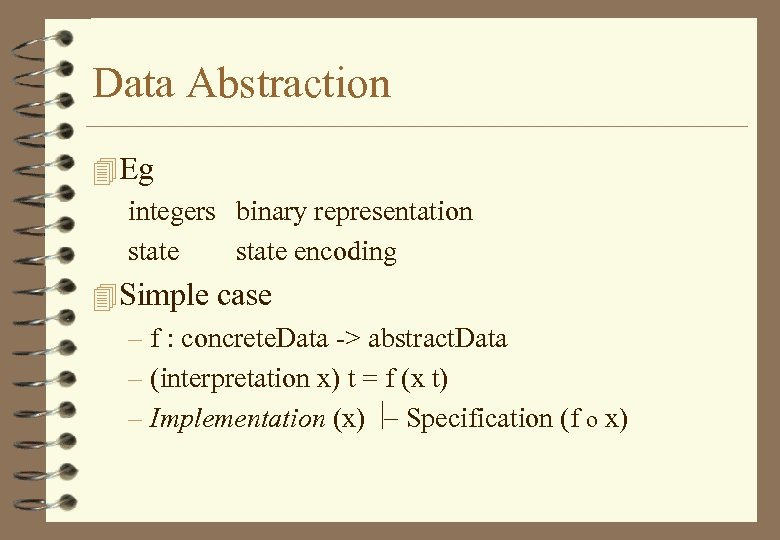Data Abstraction 4 Eg integers binary representation state encoding 4 Simple case – f : concrete. Data -> abstract. Data – (interpretation x) t = f (x t) – Implementation (x) ú- Specification (f o x)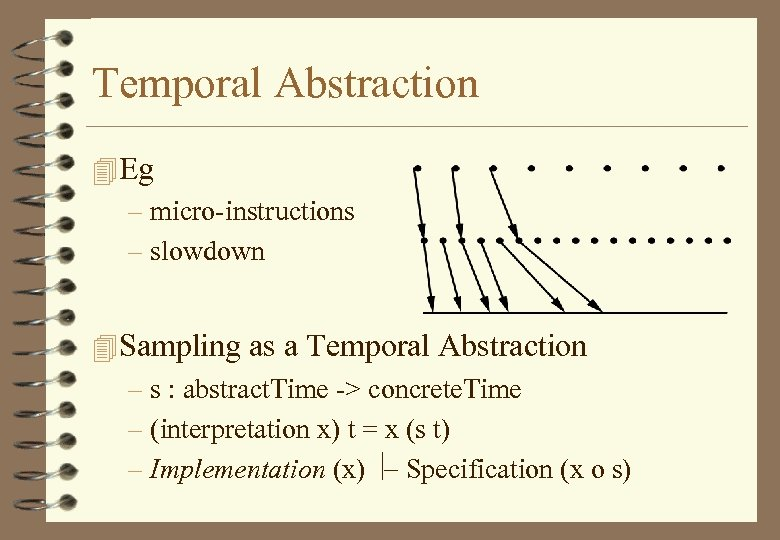Temporal Abstraction 4 Eg – micro-instructions – slowdown 4 Sampling as a Temporal Abstraction – s : abstract. Time -> concrete. Time – (interpretation x) t = x (s t) – Implementation (x) ú- Specification (x o s)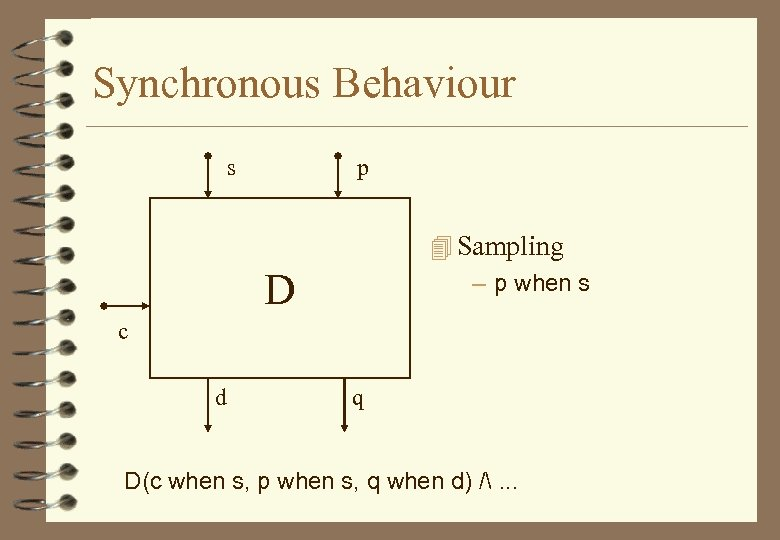Synchronous Behaviour s p 4 Sampling – p when s D c d q D(c when s, p when s, q when d) /. . .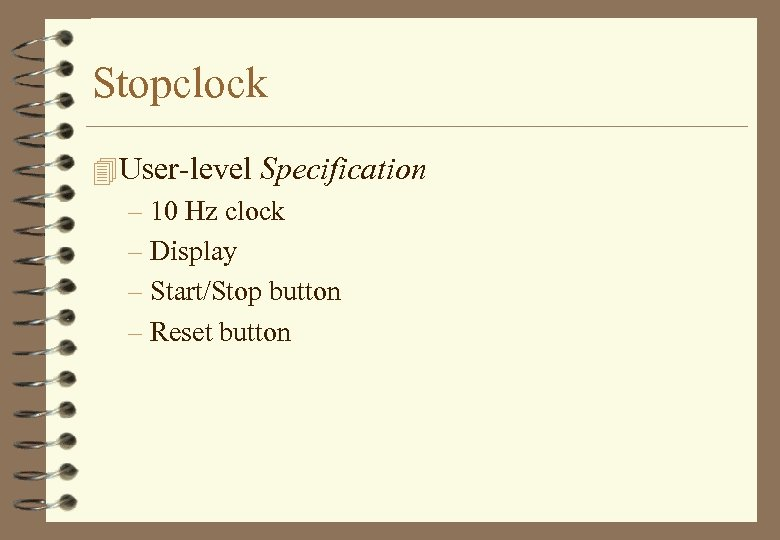Stopclock 4 User-level Specification – 10 Hz clock – Display – Start/Stop button – Reset button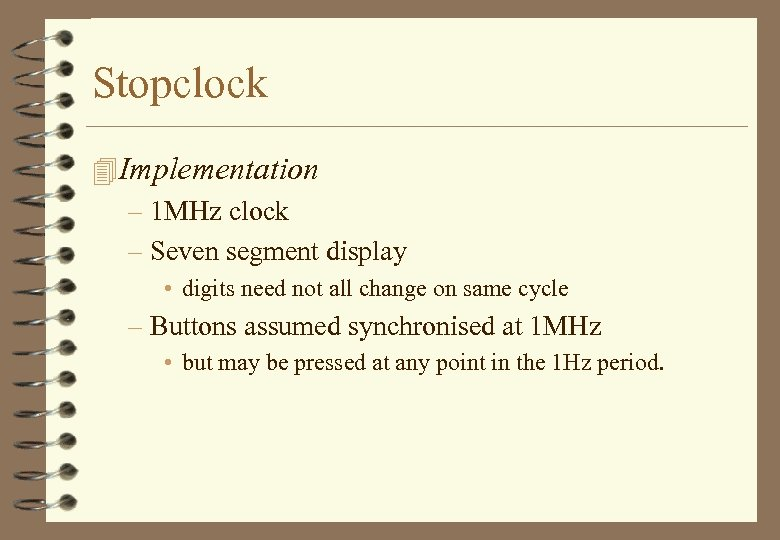Stopclock 4 Implementation – 1 MHz clock – Seven segment display • digits need not all change on same cycle – Buttons assumed synchronised at 1 MHz • but may be pressed at any point in the 1 Hz period.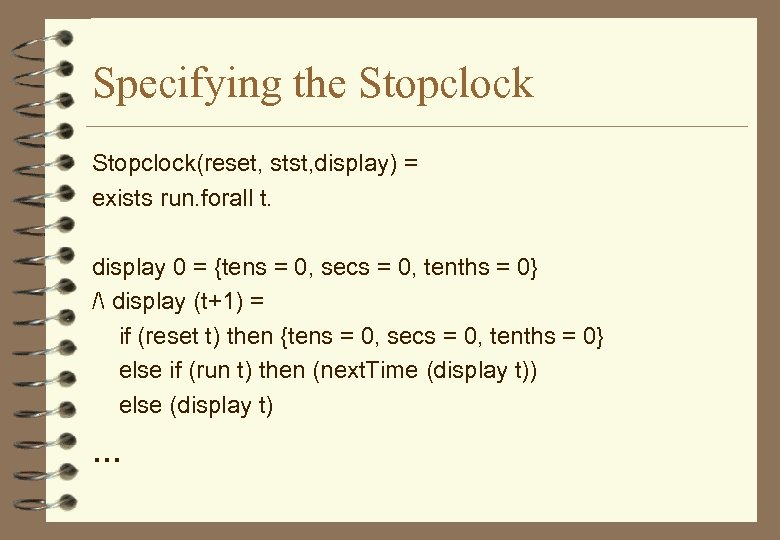Specifying the Stopclock(reset, stst, display) = exists run. forall t. display 0 = {tens = 0, secs = 0, tenths = 0} / display (t+1) = if (reset t) then {tens = 0, secs = 0, tenths = 0} else if (run t) then (next. Time (display t)) else (display t) . . .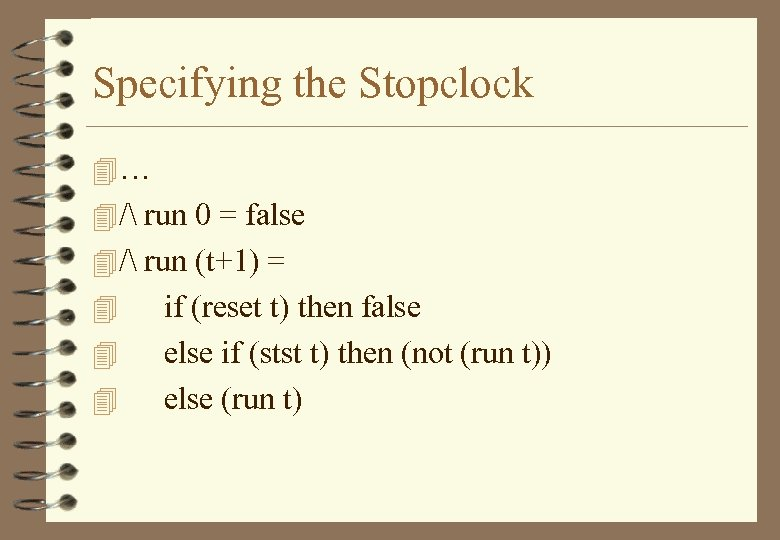Specifying the Stopclock 4… 4 / run 0 = false 4 / run (t+1) = 4 4 4 if (reset t) then false else if (stst t) then (not (run t)) else (run t)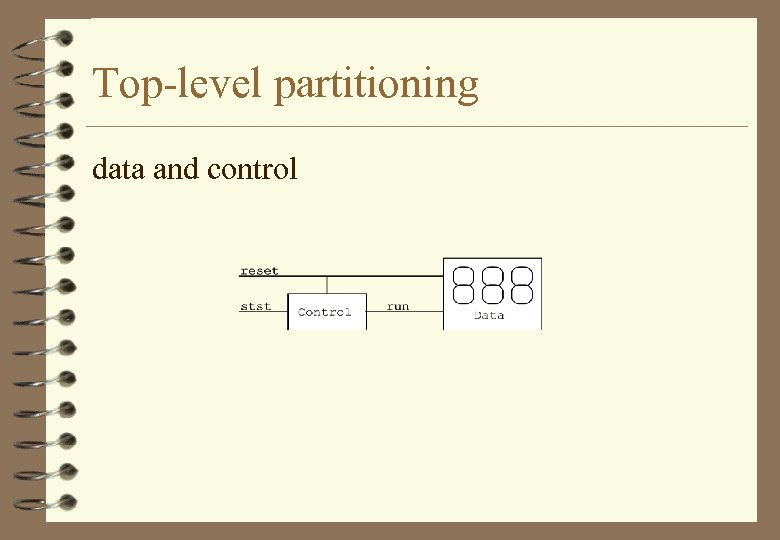Top-level partitioning data and control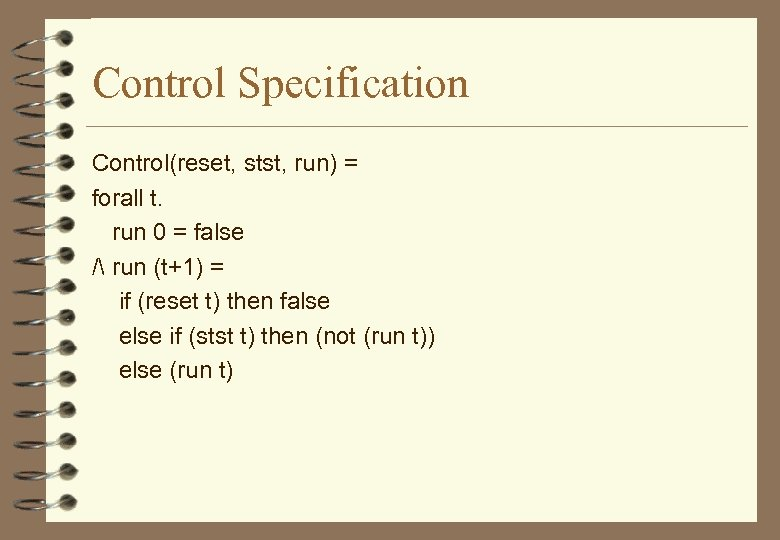Control Specification Control(reset, stst, run) = forall t. run 0 = false / run (t+1) = if (reset t) then false else if (stst t) then (not (run t)) else (run t)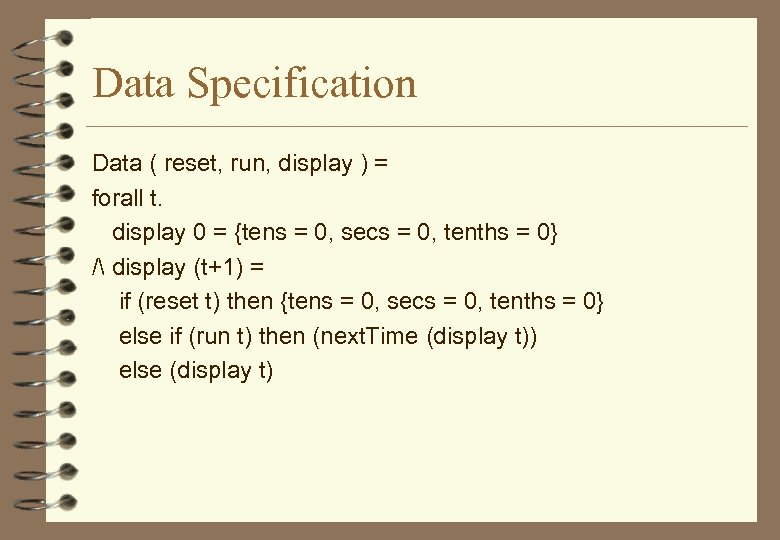Data Specification Data ( reset, run, display ) = forall t. display 0 = {tens = 0, secs = 0, tenths = 0} / display (t+1) = if (reset t) then {tens = 0, secs = 0, tenths = 0} else if (run t) then (next. Time (display t)) else (display t)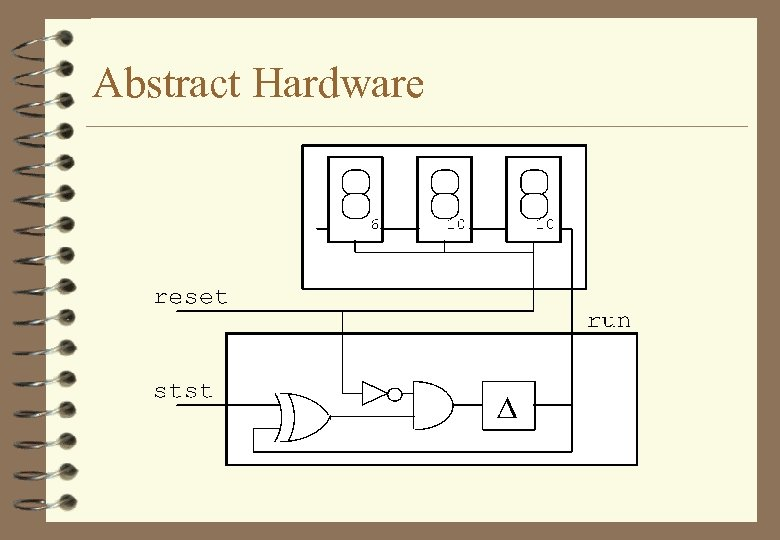Abstract Hardware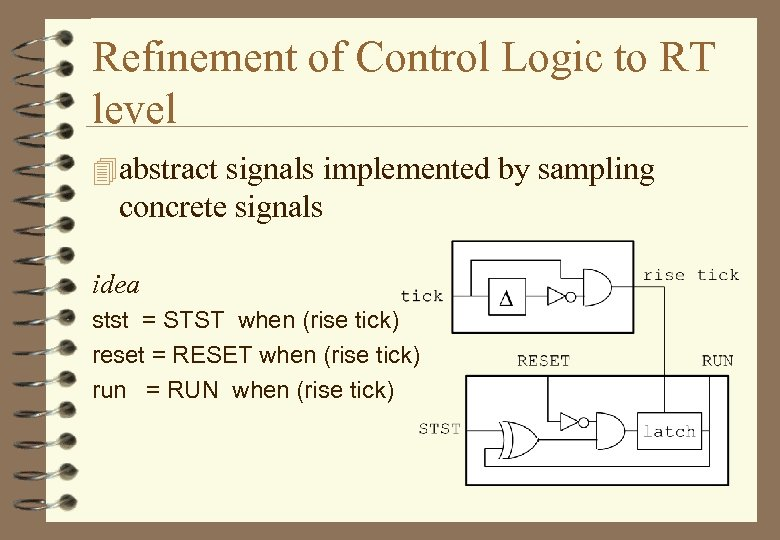Refinement of Control Logic to RT level 4 abstract signals implemented by sampling concrete signals idea stst = STST when (rise tick) reset = RESET when (rise tick) run = RUN when (rise tick)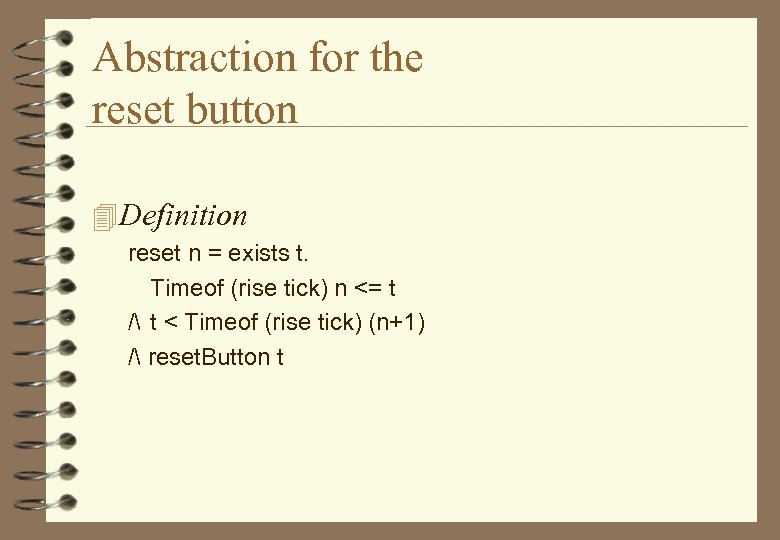Abstraction for the reset button 4 Definition reset n = exists t. Timeof (rise tick) n <= t / t < Timeof (rise tick) (n+1) / reset. Button t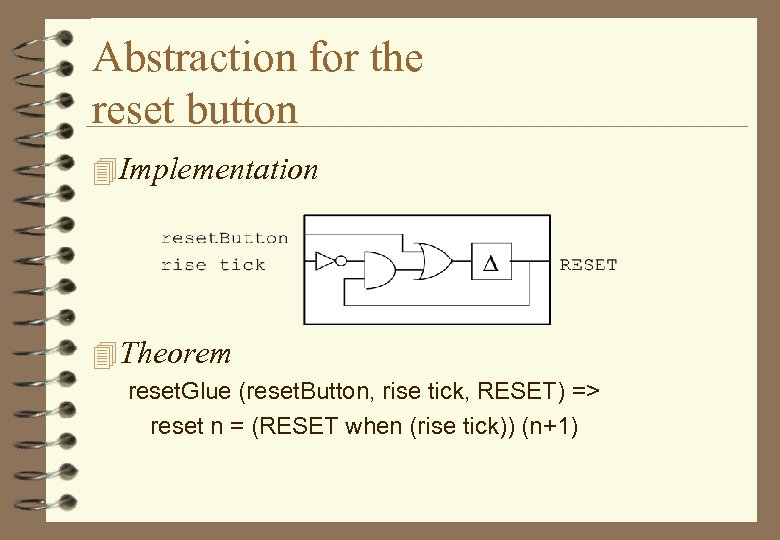Abstraction for the reset button 4 Implementation 4 Theorem reset. Glue (reset. Button, rise tick, RESET) => reset n = (RESET when (rise tick)) (n+1)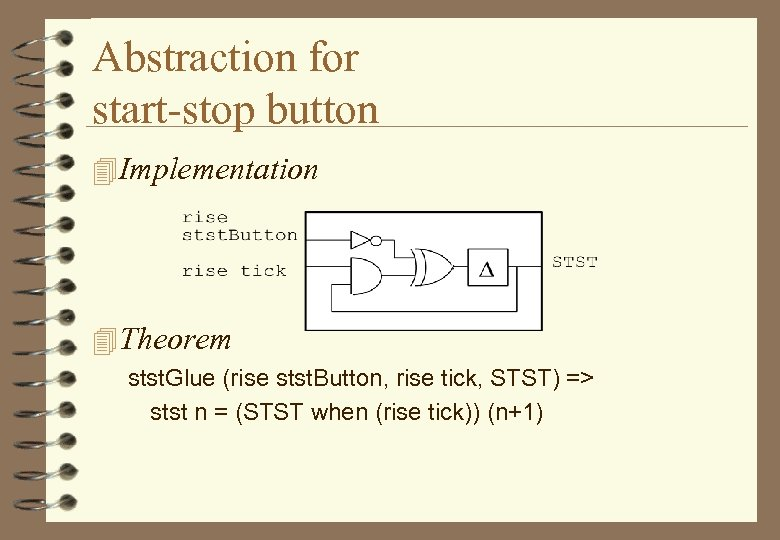Abstraction for start-stop button 4 Implementation 4 Theorem stst. Glue (rise stst. Button, rise tick, STST) => stst n = (STST when (rise tick)) (n+1)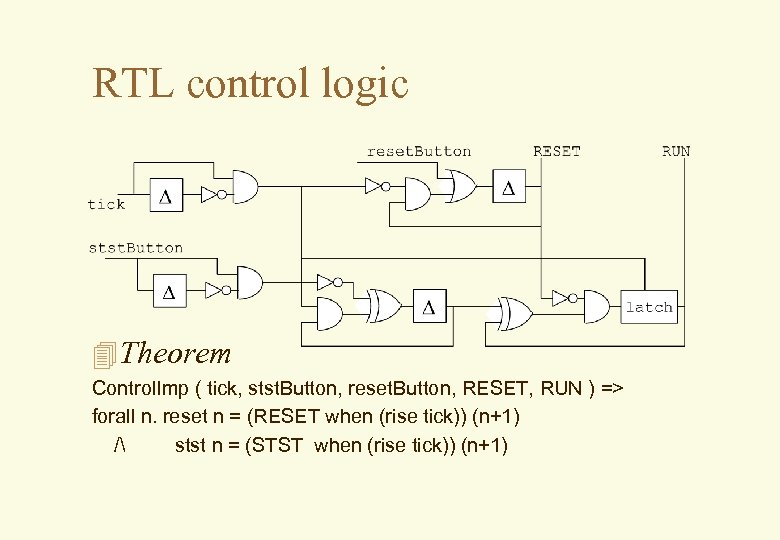RTL control logic 4 Theorem Control. Imp ( tick, stst. Button, reset. Button, RESET, RUN ) => forall n. reset n = (RESET when (rise tick)) (n+1) / stst n = (STST when (rise tick)) (n+1)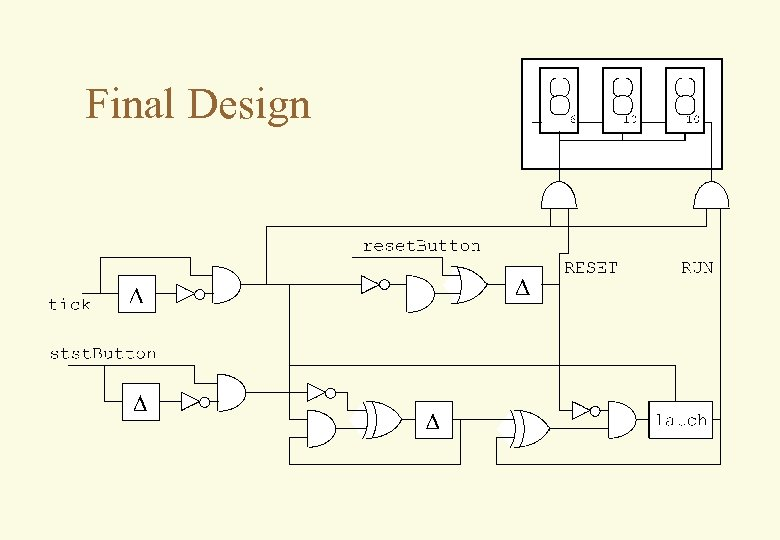Final Design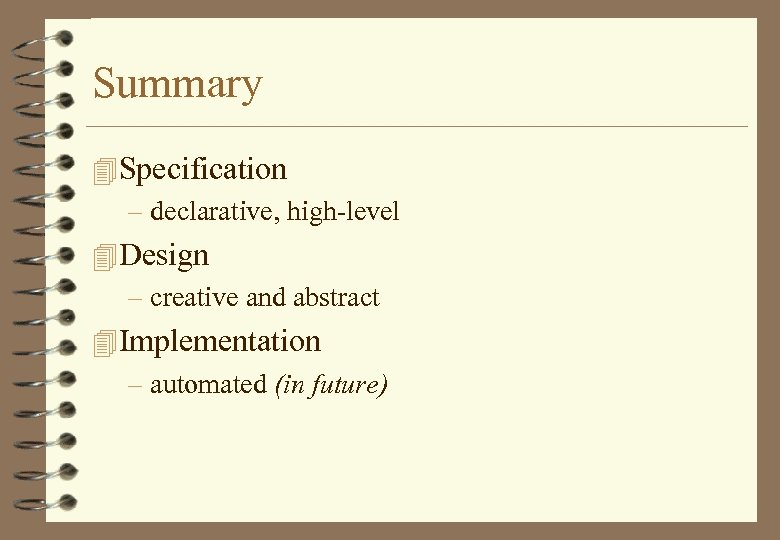Summary 4 Specification – declarative, high-level 4 Design – creative and abstract 4 Implementation – automated (in future)